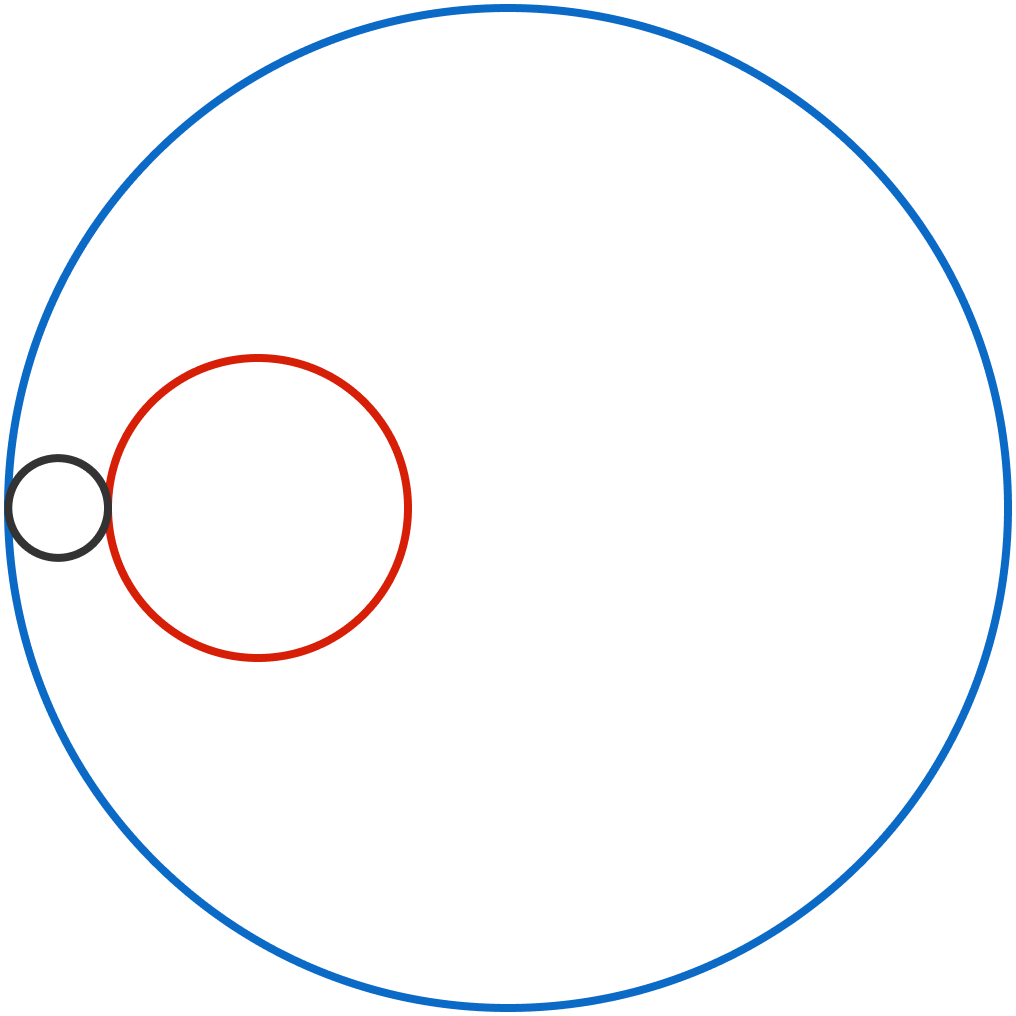# Go Round and Round

Calculus Level 3In the figure, the radii of the red circle and the blue circle are 3 and 10, respectively, and the distance between their centers is 5.

The black circle is externally tangent to the red circle and internally tangent to the blue circle. The path traced by the center of the black circles is a closed curve whose area is $X\pi.$

What is $X?$

×